# Free eBook - The Data Science Workshop - Second Edition5 (1 reviews total)
By Anthony So , Thomas V. Joseph , Robert Thas John and 2 more
• A new free eBook every day on the latest in tech
• 30 permanently free eBooks from our core tech library
Where there’s data, there’s insight. With so much data being generated, there is immense scope to extract meaningful information that’ll boost business productivity and profitability. By learning to convert raw data into game-changing insights, you’ll open new career paths and opportunities. The Data Science Workshop begins by introducing different types of projects and showing you how to incorporate machine learning algorithms in them. You’ll learn to select a relevant metric and even assess the performance of your model. To tune the hyperparameters of an algorithm and improve its accuracy, you’ll get hands-on with approaches such as grid search and random search. Next, you’ll learn dimensionality reduction techniques to easily handle many variables at once, before exploring how to use model ensembling techniques and create new features to enhance model performance. In a bid to help you automatically create new features that improve your model, the book demonstrates how to use the automated feature engineering tool. You’ll also understand how to use the orchestration and scheduling workflow to deploy machine learning models in batch. By the end of this book, you’ll have the skills to start working on data science projects confidently. By the end of this book, you’ll have the skills to start working on data science projects confidently.
Publication date:
August 2020
Publisher
Packt
Pages
824
ISBN
9781800566927

# 2. Regression

Overview

This chapter is an introduction to linear regression analysis and its application to practical problem-solving in data science. You will learn how to use Python, a versatile programming language, to carry out regression analysis and examine the results. The use of the logarithm function to transform inherently non-linear relationships between variables and to enable the application of the linear regression method of analysis will also be introduced.

By the end of this chapter, you will be able to identify and import the Python modules required for regression analysis; use the `pandas` module to load a dataset and prepare it for regression analysis; create a scatter plot of bivariate data and fit a regression line through it; use the methods available in the Python `statsmodels` module to fit a regression model to a dataset; explain the results of simple and multiple linear regression analysis; assess the goodness of fit of a linear regression model; and apply linear regression analysis as a tool for practical problem-solving.

# Introduction

The previous chapter provided a primer to Python programming and an overview of the data science field. Data science is a relatively young multidisciplinary field of study. It draws its concepts and methods from the traditional fields of statistics, computer science, and the broad field of artificial intelligence (AI), especially the subfield of AI called machine learning: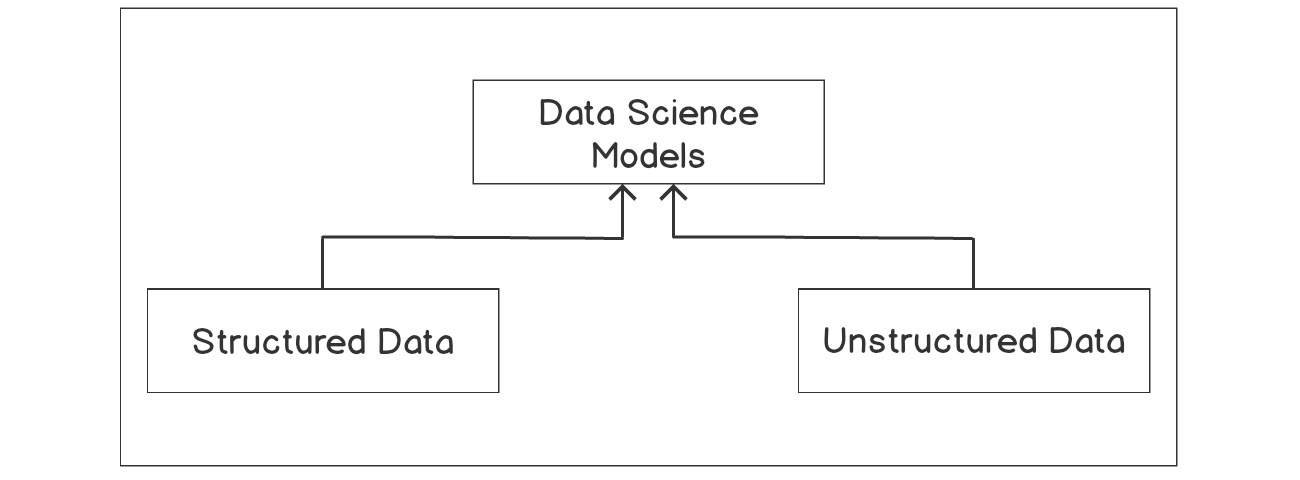Figure 2.1: The data science models

As you can see in Figure 2.1, data science aims to make use of both structured and unstructured data, develop models that can be effectively used, make predictions, and also derive insights for decision making.

A loose description of structured data will be any set of data that can be conveniently arranged into a table that consists of rows and columns. This kind of data is normally stored in database management systems.

Unstructured data, however, cannot be conveniently stored in tabular form – an example of such a dataset is a text document. To achieve the objectives of data science, a flexible programming language that effectively combines interactivity with computing power and speed is necessary. This is where the Python programming language meets the needs of data science and, as mentioned in Chapter 1, Introduction to Data Science in Python, we will be using Python in this book.

The need to develop models to make predictions and to gain insights for decisionmaking cuts across many industries. Data science is, therefore, finding uses in many industries, including healthcare, manufacturing and the process industries in general, the banking and finance sectors, marketing and e-commerce, the government, and education.

In this chapter, we will be specifically be looking at regression, which is one of the key methods that is used regularly in data science, in order to model relationships between variables, where the target variable (that is, the value you're looking for) is a real number.

Consider a situation where a real estate business wants to understand and, if possible, model the relationship between the prices of property in a city and knowing the key attributes of the properties. This is a data science problem and it can be tackled using regression.

This is because the target variable of interest, which is the price of a property, is a real number. Examples of the key attributes of a property that can be used to predict its value are as follows:

• The age of the property
• The number of bedrooms in a property
• Whether the property has a pool or not
• The area of land the property covers
• The distance of the property from facilities such as railway stations and schools

Regression analysis can be employed to study this scenario, in which you have to create a function that maps the key attributes of a property to the target variable, which, in this case, is the price of a property.

Regression analysis is part of a family of machine learning techniques called supervised machine learning. It is called supervised because the machine learning algorithm that learns the model is provided a kind of question and answer dataset to learn from. The question here is the key attribute and the answer is the property price for each property that is used in the study, as shown in the following figure:Figure 2.2: Example of a supervised learning technique

Once a model has been learned by the algorithm, we can provide the model with a question (that is, a set of attributes for a property whose price we want to find) for it to tell us what the answer (that is, the price) of that property will be.

This chapter is an introduction to linear regression and how it can be applied to solve practical problems like the one described previously in data science. Python provides a rich set of modules (libraries) that can be used to conduct rigorous regression analysis of various kinds. In this chapter, we will make use of the following Python modules, among others: `pandas`, `statsmodels`, `seaborn`, `matplotlib`, and `scikit-learn`.

# Simple Linear Regression

In Figure 2.3, you can see the crime rate per capita and the median value of owner-occupied homes for the city of Boston, which is the largest city of the Commonwealth of Massachusetts. We seek to use regression analysis to gain an insight into what drives crime rates in the city.

Such analysis is useful to policy makers and society in general because it can help with decision-making directed toward the reduction of the crime rate, and hopefully the eradication of crime across communities. This can make communities safer and increase the quality of life in society.

This is a data science problem and is of the supervised machine learning type. There is a dependent variable named `crime rate` (let's denote it Y), whose variation we seek to understand in terms of an independent variable, named `Median value of owner-occupied homes` (let's denote it X).

In other words, we are trying to understand the variation in crime rate based on different neighborhoods.

Regression analysis is about finding a function, under a given set of assumptions, that best describes the relationship between the dependent variable (Y in this case) and the independent variable (X in this case).

When the number of independent variables is only one, and the relationship between the dependent and the independent variable is assumed to be a straight line, as shown in Figure 2.3, this type of regression analysis is called simple linear regression. The straight-line relationship is called the regression line or the line of best fit: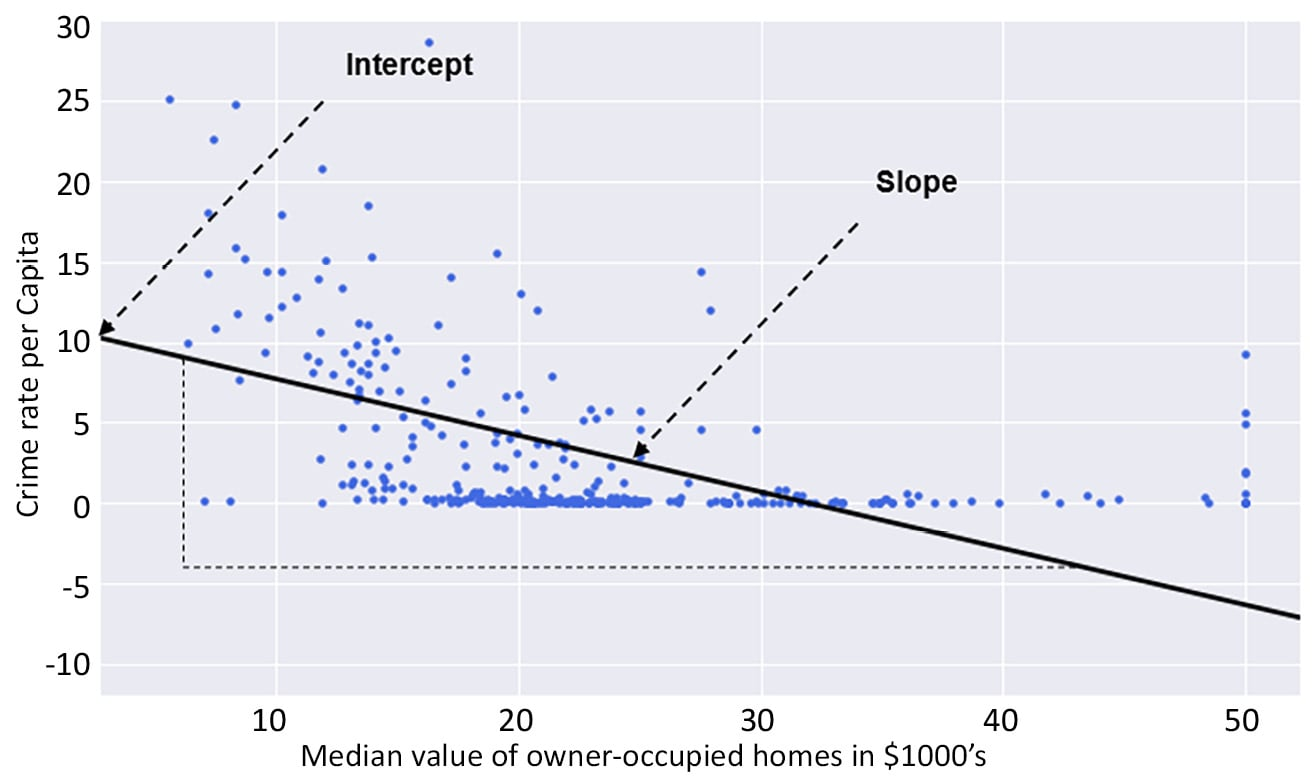Figure 2.3: A scatter plot of the crime rate against the median value of owner-occupied homes

In Figure 2.3, the regression line is shown as a solid black line. Ignoring the poor quality of the fit of the regression line to the data in the figure, we can see a decline in crime rate per capita as the median value of owner-occupied homes increases.

From a data science point of view, this observation may pose lots of questions. For instance, what is driving the decline in crime rate per capita as the median value of owner-occupier homes increases? Are richer suburbs and towns receiving more policing resources than less fortunate suburbs and towns? Unfortunately, these questions cannot be answered with such a simple plot as we find in Figure 2.3. But the observed trend may serve as a starting point for a discussion to review the distribution of police and community-wide security resources.

Returning to the question of how well the regression line fits the data, it is evident that almost one-third of the regression line has no data points scattered around it at all. Many data points are simply clustered on the horizontal axis around the zero (`0`) crime rate mark. This is not what you expect of a good regression line that fits the data well. A good regression line that fits the data well must sit amidst a cloud of data points.

It appears that the relationship between the crime rate per capita and the median value of owner-occupied homes is not as linear as you may have thought initially.

In this chapter, we will learn how to use the logarithm function (a mathematical function for transforming values) to linearize the relationship between the crime rate per capita and the median value of owner-occupied homes, in order to improve the fit of the regression line to the data points on the scatter graph.

We have ignored a very important question thus far. That is, how can you determine the regression line for a given set of data?

A common method used to determine the regression line is called the method of least squares, which is covered in the next section.

## The Method of Least Squares

The simple linear regression line is generally of the form shown in Figure 2.4, where β0 and β1 are unknown constants, representing the intercept and the slope of the regression line, respectively.

The intercept is the value of the dependent variable (Y) when the independent variable (X) has a value of zero (0). The slope is a measure of the rate at which the dependent variable (Y) changes when the independent variable (X) changes by one (1). The unknown constants are called the model coefficients or parameters. This form of the regression line is sometimes known as the population regression line, and, as a probabilistic model, it fits the dataset approximately, hence the use of the symbol (`≈`) in Figure 2.4. The model is called probabilistic because it does not model all the variability in the dependent variable (Y) :Figure 2.4: Simple linear regression equation

Calculating the difference between the actual dependent variable value and the predicted dependent variable value gives an error that is commonly termed as the residual (ϵi).

Repeating this calculation for every data point in the sample, the residual (ϵi) for every data point can be squared, to eliminate algebraic signs, and added together to obtain the error sum of squares (ESS).

The least squares method seeks to minimize the ESS.

# Multiple Linear Regression

In the simple linear regression discussed previously, we only have one independent variable. If we include multiple independent variables in our analysis, we get a multiple linear regression model. Multiple linear regression is represented in a way that's similar to simple linear regression.

Let's consider a case where we want to fit a linear regression model that has three independent variables, X1, X2, and X3. The formula for the multiple linear regression equation will look like Figure 2.5:Figure 2.5: Multiple linear regression equation

Each independent variable will have its own coefficient or parameter (that is, β1 β2 or β3). The βs coefficient tells us how a change in their respective independent variable influences the dependent variable if all other independent variables are unchanged.

## Estimating the Regression Coefficients (β0, β1, β2 and β3)

The regression coefficients in Figure 2.5 are estimated using the same least squares approach that was discussed when simple linear regression was introduced. To satisfy the least squares method, the chosen coefficients must minimize the sum of squared residuals.

Later in the chapter, we will make use of the Python programming language to compute these coefficient estimates practically.

## Logarithmic Transformations of Variables

As has been mentioned already, sometimes the relationship between the dependent and independent variables is not linear. This limits the use of linear regression. To get around this, depending on the nature of the relationship, the logarithm function can be used to transform the variable of interest. What happens then is that the transformed variable tends to have a linear relationship with the other untransformed variables, enabling the use of linear regression to fit the data. This will be illustrated in practice on the dataset being analyzed later in the exercises of the book.

## Correlation Matrices

In Figure 2.3, we saw how a linear relationship between two variables can be analyzed using a straight-line graph. Another way of visualizing the linear relationship between variables is with a correlation matrix. A correlation matrix is a kind of cross-table of numbers showing the correlation between pairs of variables, that is, how strongly the two variables are connected (this can be thought of as how a change in one variable will cause a change in the other variable). It is not easy analyzing raw figures in a table. A correlation matrix can, therefore, be converted to a form of "heatmap" so that the correlation between variables can easily be observed using different colors. An example of this is shown in Exercise 2.01, Loading and Preparing the Data for Analysis.

# Conducting Regression Analysis Using Python

Having discussed the basics of regression analysis, it is now time to get our hands dirty and actually do some regression analysis using Python.

To begin with our analysis, we need to start a session in Python and load the relevant modules and dataset required.

All of the regression analysis we will do in this chapter will be based on the Boston Housing dataset. The dataset is good for teaching and is suitable for linear regression analysis. It presents the level of challenge that necessitates the use of the logarithm function to transform variables in order to achieve a better level of model fit to the data. The dataset contains information on a collection of properties in the Boston area and can be used to determine how the different housing attributes of a specific property affect the property's value.

The column headings of the Boston Housing dataset CSV file can be explained as follows:

• CRIM – per capita crime rate by town
• ZN – proportion of residential land zoned for lots over 25,000 sq.ft.
• INDUS – proportion of non-retail business acres per town
• CHAS – Charles River dummy variable (= 1 if tract bounds river; 0 otherwise)
• NOX – nitric oxide concentration (parts per 10 million)
• RM – average number of rooms per dwelling
• AGE – proportion of owner-occupied units built prior to 1940
• DIS – weighted distances to five Boston employment centers
• TAX – full-value property-tax rate per \$10,000
• PTRATIO – pupil-teacher ratio by town
• LSTAT – % of lower status of the population
• MEDV – median value of owner-occupied homes in \$1,000s

The dataset we're using is a slightly modified version of the original and was sourced from https://packt.live/39IN8Y6.

In this exercise, we will learn how to load Python modules, and the dataset we need for analysis, into our Python session and prepare the data for analysis.

Note

We will be using the Boston Housing dataset in this chapter, which can be found on our GitHub repository at https://packt.live/2QCCbQB.

1. Open a new Colab notebook file.
2. Load the necessary Python modules by entering the following code snippet into a single Colab notebook cell. Press the Shift and Enter keys together to run the block of code:
```%matplotlib inline
import matplotlib as mpl
import seaborn as sns
import matplotlib.pyplot as plt
import statsmodels.formula.api as smf
import statsmodels.graphics.api as smg
import pandas as pd
import numpy as np
import patsy
from statsmodels.graphics.correlation import plot_corr
from sklearn.model_selection import train_test_split
plt.style.use('seaborn')```

The first line of the preceding code enables `matplotlib` to display the graphical output of the code in the notebook environment. The lines of code that follow use the `import` keyword to load various Python modules into our programming environment. This includes `patsy`, which is a Python module. Some of the modules are given aliases for easy referencing, such as the `seaborn` module being given the alias `sns`. Therefore, whenever we refer to `seaborn` in subsequent code, we use the alias `sns`. The `patsy` module is imported without an alias. We, therefore, use the full name of the `patsy` module in our code where needed.

The `plot_corr` and `train_test_split` functions are imported from the `statsmodels.graphics.correlation` and `sklearn.model_selection` modules respectively. The last statement is used to set the aesthetic look of the graphs that `matplotlib` generates to the type displayed by the `seaborn` module.

3. Next, load the `Boston.CSV` file and assign the variable name `rawBostonData` to it by running the following code:
```rawBostonData = pd.read_csv\
('https://raw.githubusercontent.com/'\
'PacktWorkshops/The-Data-Science-'\
'Workshop/master/Chapter02/'\
'Dataset/Boston.csv')```
4. Inspect the first five records in the DataFrame:
`rawBostonData.head()`

You should get the following output: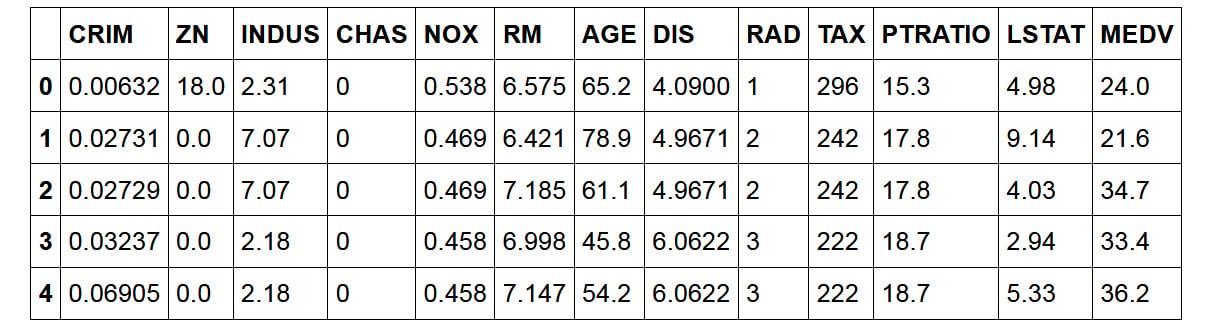Figure 2.6: First five rows of the dataset

5. Check for missing values (null values) in the DataFrame and then drop them in order to get a clean dataset Use the pandas method `dropna()` to find and remove these missing values:
`rawBostonData = rawBostonData.dropna()`
6. Check for duplicate records in the DataFrame and then drop them in order to get a clean dataset. Use the `drop_duplicates()` method from pandas:
`rawBostonData = rawBostonData.drop_duplicates()`
7. List the column names of the DataFrame so that you can examine the fields in your dataset, and modify the names, if necessary, to names that are meaningful:
`list(rawBostonData.columns)`

You should get the following output: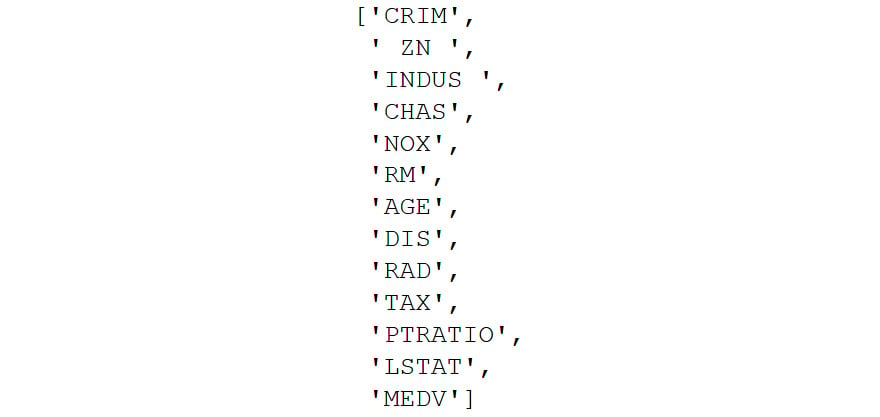Figure 2.7: Listing all the column names

8. Rename the DataFrame columns so that they are meaningful. Be mindful to match the column names exactly as leaving out even white spaces in the name strings will result in an error. For example, this string, `ZN`, has a white space before and after and it is different from `ZN`. After renaming, print the head of the new DataFrame as follows:
```renamedBostonData = rawBostonData.rename\
(columns = {\
'CRIM':'crimeRatePerCapita',\
' ZN ':'landOver25K_sqft',\
'INDUS ':'non-retailLandProptn',\
'CHAS':'riverDummy',\
'NOX':'nitrixOxide_pp10m',\
'RM':'AvgNo.RoomsPerDwelling',\
'AGE':'ProptnOwnerOccupied',\
'DIS':'weightedDist',\
'TAX':'propTaxRate_per10K',\
'PTRATIO':'pupilTeacherRatio',\
'LSTAT':'pctLowerStatus',\
'MEDV':'medianValue_Ks'})

You should get the following output: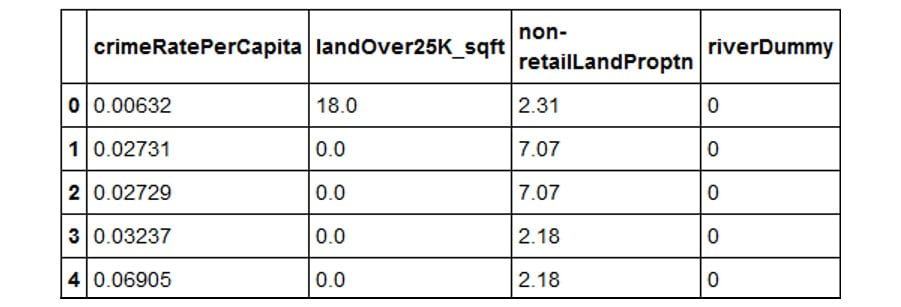Figure 2.8: DataFrames being renamed

Note

The preceding output is truncated. Please head to the GitHub repository to find the entire output.

9. Inspect the data types of the columns in your DataFrame using the `.info()` function:
`renamedBostonData.info()`

You should get the following output: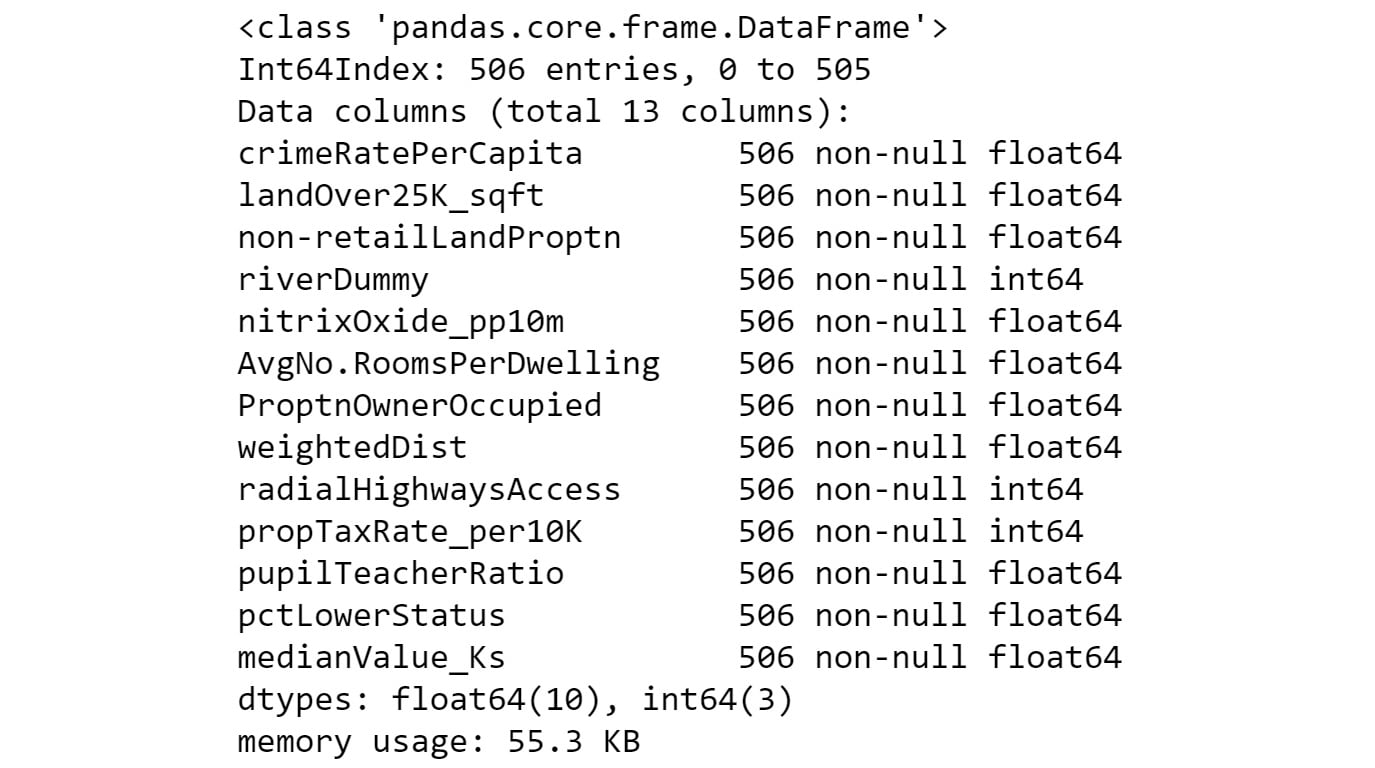Figure 2.9: The different data types in the dataset

The output shows that there are `506` rows (`Int64Index: 506 entries`) in the dataset. There are also `13` columns in total (`Data columns`). None of the `13` columns has a row with a missing value (all `506` rows are non-null). 10 of the columns have floating-point (decimal) type data and three have integer type data.

10. Now, calculate basic statistics for the numeric columns in the DataFrame:
`renamedBostonData.describe(include=[np.number]).T`

We used the pandas function, `describe`, called on the DataFrame to calculate simple statistics for numeric fields (this includes any field with a `numpy` number data type) in the DataFrame. The statistics include the minimum, the maximum, the count of rows in each column, the average of each column (mean), the 25th percentile, the 50th percentile, and the 75th percentile. We transpose (using the `.T` function) the output of the `describe` function to get a better layout.

You should get the following output: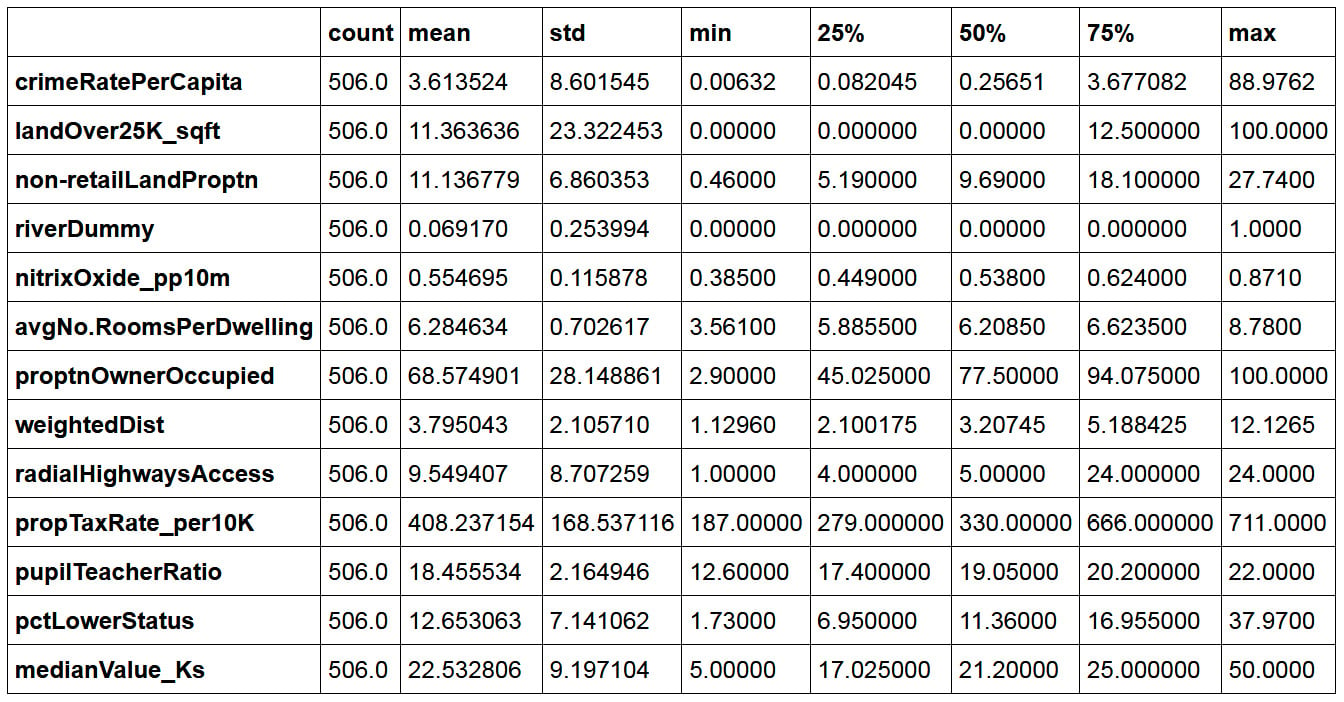Figure 2.10: Basic statistics of the numeric column

11. Divide the DataFrame into training and test sets, as shown in the following code snippet:
```X = renamedBostonData.drop('crimeRatePerCapita', axis = 1)
y = renamedBostonData[['crimeRatePerCapita']]
seed = 10
test_data_size = 0.3
X_train, X_test, \
y_train, y_test = train_test_split(X, y, \
test_size = test_data_size, \
random_state = seed)
train_data = pd.concat([X_train, y_train], axis = 1)
test_data = pd.concat([X_test, y_test], axis = 1)```

We choose a test data size of 30%, which is `0.3`. The `train_test_split` function is used to achieve this. We set the seed of the random number generator so that we can obtain a reproducible split each time we run this code. An arbitrary value of `10` is used here. It is good model-building practice to divide a dataset being used to develop a model into at least two parts. One part is used to develop the model and it is called a training set (`X_train` and `y_train` combined).

Note

Splitting your data into training and test subsets allows you to use some of the data to train your model (that is, it lets you build a model that learns the relationships between the variables), and the rest of the data to test your model (that is, to see how well your new model can make predictions when given new data). You will use train-test splits throughout this book, and the concept will be explained in more detail in Chapter 7, The Generalization Of Machine Learning Models.

12. Calculate and plot a correlation matrix for the `train_data` set:
```corrMatrix = train_data.corr(method = 'pearson')
xnames=list(train_data.columns)
ynames=list(train_data.columns)
plot_corr(corrMatrix, xnames=xnames, ynames=ynames,\
title=None, normcolor=False, cmap='RdYlBu_r')```

The use of the backslash character, `\`, on line 4 in the preceding code snippet is to enforce the continuation of code on to a new line in Python. The `\` character is not required if you are entering the full line of code into a single line in your notebook.

You should get the following output: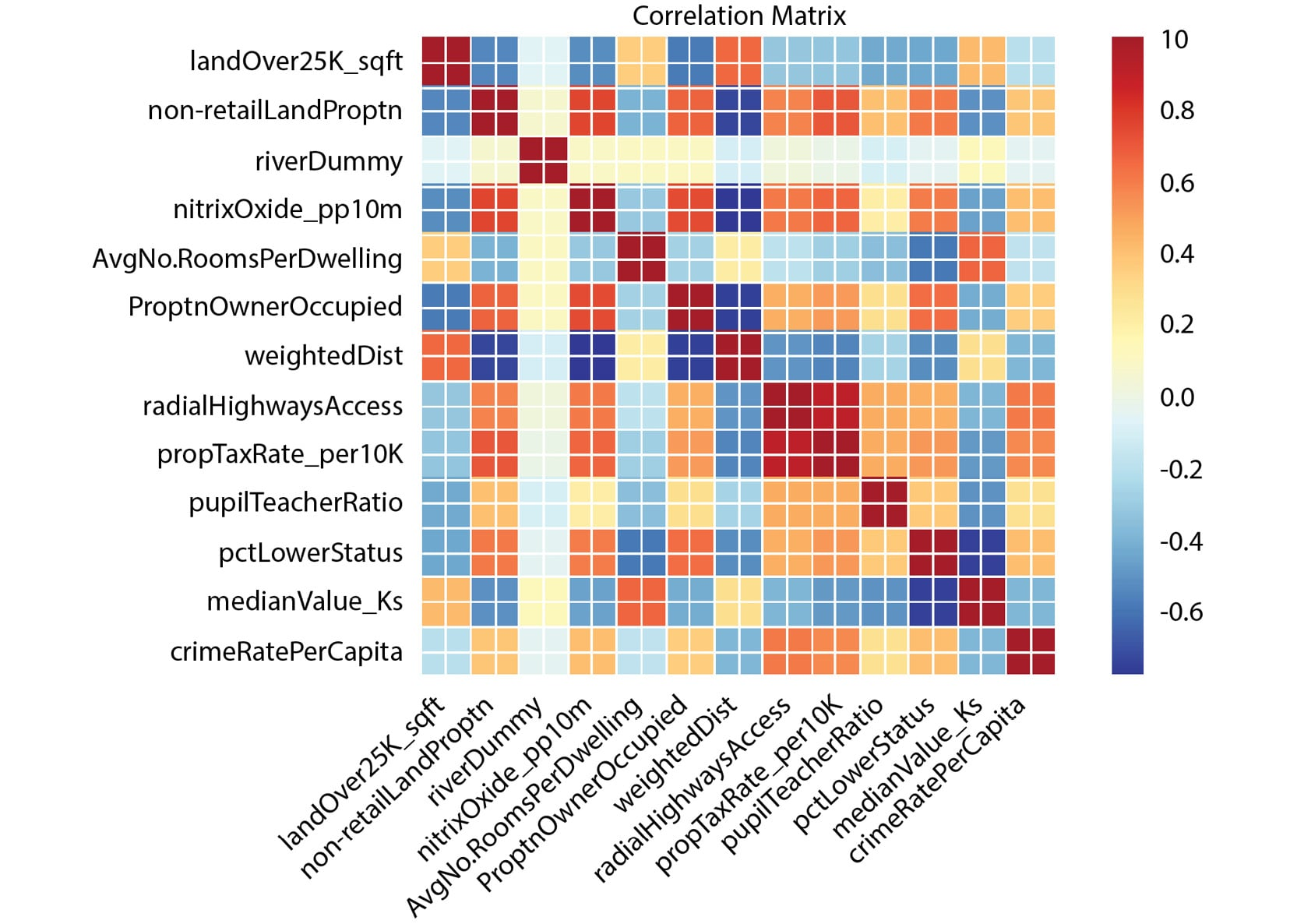Figure 2.11: Output with the expected heatmap

In the preceding heatmap, we can see that there is a strong positive correlation (an increase in one causes an increase in the other) between variables that have orange or red squares. There is a strong negative correlation (an increase in one causes a decrease in the other) between variables with blue squares. There is little or no correlation between variables with pale-colored squares. For example, there appears to be a relatively strong correlation between `nitrixOxide_pp10m` and `non-retailLandProptn`, but a low correlation between `riverDummy` and any other variable.

Note

To access the source code for this specific section, please refer to https://packt.live/320HLAH.

You can also run this example online at https://packt.live/34baJAA.

We can use the findings from the correlation matrix as the starting point for further regression analysis. The heatmap gives us a good overview of relationships in the data and can show us which variables to target in our investigation.

## The Correlation Coefficient

In the previous exercise, we have seen how a correlation matrix heatmap can be used to visualize the relationships between pairs of variables. We can also see these same relationships in numerical form using the raw correlation coefficient numbers. These are values between -1 and 1, which represent how closely two variables are linked.

Pandas provides a `corr` function, which when called on DataFrame provides a matrix (table) of the correlation of all numeric data types. In our case, running the code, `train_data.corr (method = 'pearson')`, in the Colab notebook provides the results in Figure 2.12.

Note

Pearson is the standard correlation coefficient for measuring the relationship between variables.

It is important to note that Figure 2.12 is symmetric along the left diagonal. The left diagonal values are correlation coefficients for features against themselves (and so all of them have a value of one (1)), and therefore are not relevant to our analysis. The data in Figure 2.12 is what is presented as a plot in the output of Step 12 in Exercise 2.01, Loading and Preparing the Data for Analysis.

You should get the following output: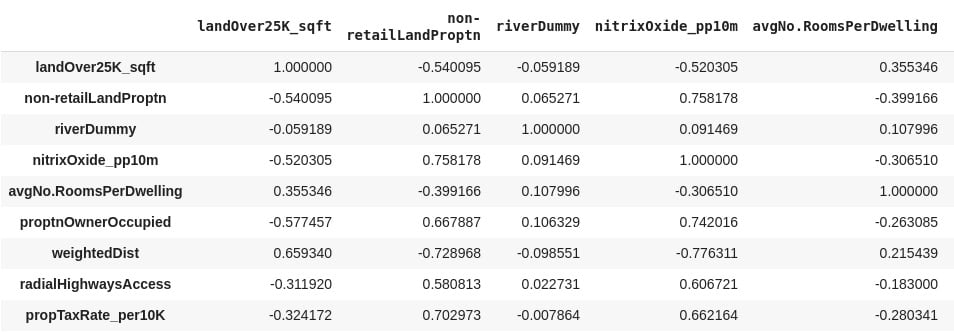Figure 2.12: A correlation matrix of the training dataset

Note

The preceding output is truncated.

Data scientists use the correlation coefficient as a statistic in order to measure the linear relationship between two numeric variables, X and Y. The correlation coefficient for a sample of bivariate data is commonly represented by r. In statistics, the common method to measure the correlation between two numeric variables is by using the Pearson correlation coefficient. Going forward in this chapter, therefore, any reference to the correlation coefficient means the Pearson correlation coefficient.

To practically calculate the correlation coefficient statistic for the variables in our dataset in this course, we use a Python function. What is important to this discussion is the meaning of the values the correlation coefficient we calculate takes. The correlation coefficient (r) takes values between +1 and -1.

When r is equal to +1, the relationship between X and Y is such that both X and Y increase or decrease in the same direction perfectly. When r is equal to -1, the relationship between X and Y is such that an increase in X is associated with a decrease in Y perfectly and vice versa. When r is equal to zero (0), there is no linear relationship between X and Y.

Having no linear relationship between X and Y does not mean that X and Y are not related; instead, it means that if there is any relationship, it cannot be described by a straight line. In practice, correlation coefficient values around 0.6 or higher (or -0.6 or lower) is a sign of a potentially exciting linear relationship between two variables, X and Y.

The last column of the output of Exercise 2.01, Loading and Preparing the Data for Analysis, Step 12, provides r values for crime rate per capita against other features in color shades. Using the color bar, it is obvious that `radialHighwaysAccess`, `propTaxRate_per10K`, `nitrixOxide_pp10m`, and `pctLowerStatus` have the strongest correlation with crime rate per capita. This indicates that a possible linear relationship, between crime rate per capita and any of these independent variables, may be worth looking into.

## Exercise 2.02: Graphical Investigation of Linear Relationships Using Python

Scatter graphs fitted with a regression line are a quick way by which a data scientist can visualize a possible correlation between a dependent variable and an independent variable.

The goal of the exercise is to use this technique to investigate any linear relationship that may exist between crime rate per capita and the median value of owner-occupied homes in towns in the city of Boston.

1. Open a new Colab notebook file and execute the steps up to and including Step 11 from Exercise 2.01, Loading and Preparing the Data for Analysis. This is shown in the code blocks below, starting with the import statements:
```%matplotlib inline
import matplotlib as mpl
import seaborn as sns
import matplotlib.pyplot as plt
import statsmodels.formula.api as smf
import statsmodels.graphics.api as smg
import pandas as pd
import numpy as np
import patsy
from statsmodels.graphics.correlation import plot_corr
from sklearn.model_selection import train_test_split
plt.style.use('seaborn')```

```rawBostonData = pd.read_csv\
('https://raw.githubusercontent.com/'\
'PacktWorkshops/The-Data-Science-'\
'Workshop/master/Chapter02/'
'Dataset/Boston.csv')
rawBostonData = rawBostonData.dropna()
rawBostonData = rawBostonData.drop_duplicates()
renamedBostonData = rawBostonData.rename\
(columns = {\
'CRIM':'crimeRatePerCapita',\
' ZN ':'landOver25K_sqft',\
'INDUS ':'non-retailLandProptn',\
'CHAS':'riverDummy',\
'NOX':'nitrixOxide_pp10m',\
'RM':'AvgNo.RoomsPerDwelling',\
'AGE':'ProptnOwnerOccupied',\
'DIS':'weightedDist',\
'TAX':'propTaxRate_per10K',\
'PTRATIO':'pupilTeacherRatio',\
'LSTAT':'pctLowerStatus',\
'MEDV':'medianValue_Ks'})```

Setting up the test and train data:

```X = renamedBostonData.drop('crimeRatePerCapita', axis = 1)
y = renamedBostonData[['crimeRatePerCapita']]
seed = 10
test_data_size = 0.3
X_train, X_test, y_train, y_test = train_test_split\
(X, y, \
test_size = test_data_size,\
random_state = seed)
train_data = pd.concat([X_train, y_train], axis = 1)
test_data = pd.concat([X_test, y_test], axis = 1)```
2. Now use the `subplots` function in `matplotlib` to define a canvas (assigned the variable name `fig` in the following code) and a graph object (assigned the variable name `ax` in the following code) in Python. You can set the size of the graph by setting the `figsize` (width = `10`, height = `6`) argument of the function:
`fig, ax = plt.subplots(figsize=(10, 6))`

Do not execute the code yet.

3. Use the `seaborn` function `regplot` to create the scatter plot. Do not execute this code cell yet; we will add more code to style the plot in the next step:
```sns.regplot(x='medianValue_Ks', y='crimeRatePerCapita', \
ci=None, data=train_data, ax=ax, color='k', \
scatter_kws={"s": 20,"color": "royalblue", \
"alpha":1})```

The function accepts arguments for the independent variable (`x`), the dependent variable (`y`), the confidence interval of the regression parameters (`ci`), which takes values from 0 to 100, the DataFrame that has `x` and `y` (`data`), a matplotlib graph object (`ax`), and others to control the aesthetics of the points on the graph. (In this case, the confidence interval is set to `None` – we will see more on confidence intervals later in the chapter.)

4. In the same cell as step 3, set the `x` and `y` labels, the `fontsize` and `name` labels, the `x` and `y` limits, and the `tick` parameters of the matplotlib graph object (`ax`). Also, set the layout of the canvas to `tight`:
```ax.set_ylabel('Crime rate per Capita', fontsize=15, \
fontname='DejaVu Sans')
ax.set_xlabel("Median value of owner-occupied homes "\
"in \$1000's", fontsize=15, \
fontname='DejaVu Sans')
ax.set_xlim(left=None, right=None)
ax.set_ylim(bottom=None, top=30)
ax.tick_params(axis='both', which='major', labelsize=12)
fig.tight_layout()```

Now execute the cell. You should get the following output: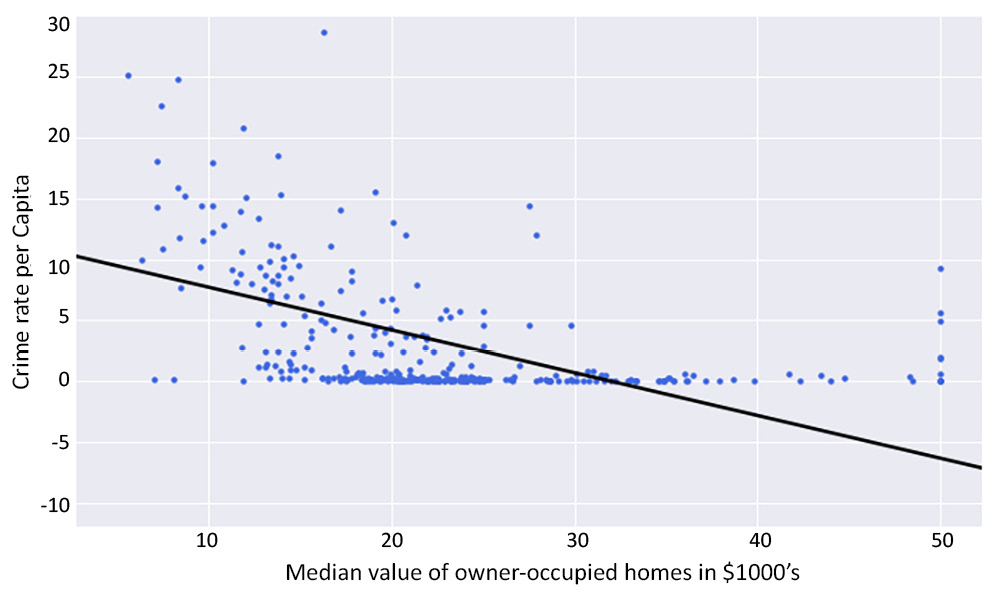Figure 2.13: Scatter graph with a regression line using Python

If the exercise was followed correctly, the output must be the same as the graph in Figure 2.3. In Figure 2.3, this output was presented and used to introduce linear regression without showing how it was created. What this exercise has taught us is how to create a scatter graph and fit a regression line through it using Python.

Note

To access the source code for this specific section, please refer to https://packt.live/34cITnk.

You can also run this example online at https://packt.live/2CA4NFG.

## Exercise 2.03: Examining a Possible Log-Linear Relationship Using Python

In this exercise, we will use the logarithm function to transform variables and investigate whether this helps provide a better fit of the regression line to the data. We will also look at how to use confidence intervals by including a 95% confidence interval of the regression coefficients on the plot.

1. Open a new Colab notebook file and execute all the steps up to Step 11 from Exercise 2.01, Loading and Preparing the Data for Analysis.
2. Use the `subplots` function in `matplotlib` to define a canvas and a graph object in Python:
`fig, ax = plt.subplots(figsize=(10, 6))`

Do not execute this code yet.

3. In the same code cell, use the logarithm function in `numpy` (`np.log`) to transform the dependent variable (`y`). This essentially creates a new variable, `log(y)`:
`y = np.log(train_data['crimeRatePerCapita'])`

Do not execute this code yet.

4. Use the seaborn `regplot` function to create the scatter plot. Set the `regplot` function confidence interval argument (`ci`) to `95%`. This will calculate a `95%` confidence interval for the regression coefficients and have them plotted on the graph as a shaded area along the regression line.

Note

A confidence interval gives an estimated range that is likely to contain the true value that you're looking for. So, a `95%` confidence interval indicates we can be `95%` certain that the true regression coefficients lie in that shaded area.

Parse the `y` argument with the new variable we defined in the preceding step. The `x` argument is the original variable from the DataFrame without any transformation. Continue in the same code cell. Do not execute this cell yet; we will add in more styling code in the next step.

```sns.regplot(x='medianValue_Ks', y=y, ci=95, \
data=train_data, ax=ax, color='k', \
scatter_kws={"s": 20,"color": "royalblue", \
"alpha":1})```
5. Continuing in the same cell, set the `x` and `y` labels, the `fontsize` and `name` labels, the `x` and `y` limits, and the `tick` parameters of the `matplotlib` graph object (`ax`). Also, set the layout of the canvas to `tight`:
```ax.set_ylabel('log of Crime rate per Capita', \
fontsize=15, fontname='DejaVu Sans')
ax.set_xlabel("Median value of owner-occupied homes "\
"in \$1000's", fontsize=15, \
fontname='DejaVu Sans')
ax.set_xlim(left=None, right=None)
ax.set_ylim(bottom=None, top=None)
ax.tick_params(axis='both', which='major', labelsize=12)
fig.tight_layout()```

Now execute this cell. The output is as follows: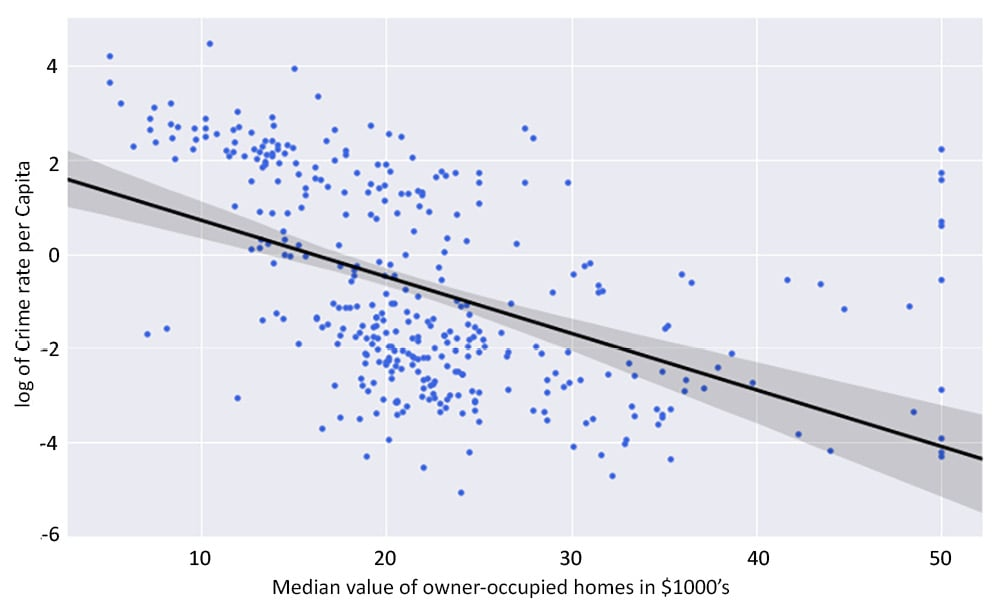Figure 2.14: Expected scatter plot with an improved linear regression line

By completing this exercise, we have successfully improved our scatter plot. The regression line created in this activity fits the data better than what was created in Exercise 2.02, Graphical Investigation of Linear Relationships Using Python. You can see by comparing the two graphs, the regression line in the log graph more clearly matches the spread of the data points. We have solved the issue where the bottom third of the line had no points clustered around it. This was achieved by transforming the dependent variable with the logarithm function. The transformed dependent variable (log of crime rate per capita) has an improved linear relationship with the median value of owner-occupied homes than the untransformed variable.

Note

To access the source code for this specific section, please refer to https://packt.live/3ay4aZS.

You can also run this example online at https://packt.live/324nN7R.

## The Statsmodels formula API

In Figure 2.3, a solid line represents the relationship between the crime rate per capita and the median value of owner-occupied homes. But how can we obtain the equation that describes this line? In other words, how can we find the intercept and the slope of the straight-line relationship?

Python provides a rich Application Programming Interface (API) for doing this easily. The statsmodels formula API enables the data scientist to use the formula language to define regression models that can be found in statistics literature and many dedicated statistical computer packages.

## Exercise 2.04: Fitting a Simple Linear Regression Model Using the Statsmodels formula API

In this exercise, we examine a simple linear regression model where the crime rate per capita is the dependent variable and the median value of owner-occupied homes is the independent variable. We use the statsmodels formula API to create a linear regression model for Python to analyze.

1. Open a new Colab notebook file and import the required packages.
```import pandas as pd
import statsmodels.formula.api as smf
from sklearn.model_selection import train_test_split```
2. Execute Step 2 to 11 from Exercise 2.01, Loading and Preparing the Data for Analysis.
3. Define a linear regression model and assign it to a variable named `linearModel`:
```linearModel = smf.ols\
(formula='crimeRatePerCapita ~ medianValue_Ks',\
data=train_data)```

As you can see, we call the `ols` function of the statsmodels API and set its formula argument by defining a `patsy` formula string that uses the tilde (`~`) symbol to relate the dependent variable to the independent variable. Tell the function where to find the variables named, in the string, by assigning the data argument of the `ols` function to the DataFrame that contains your variables (`train_data`).

4. Call the .`fit` method of the model instance and assign the results of the method to a `linearModelResult` variable, as shown in the following code snippet:
`linearModelResult = linearModel.fit()`
5. Print a summary of the results stored the `linearModelResult` variable by running the following code:
`print(linearModelResult.summary())`

You should get the following output: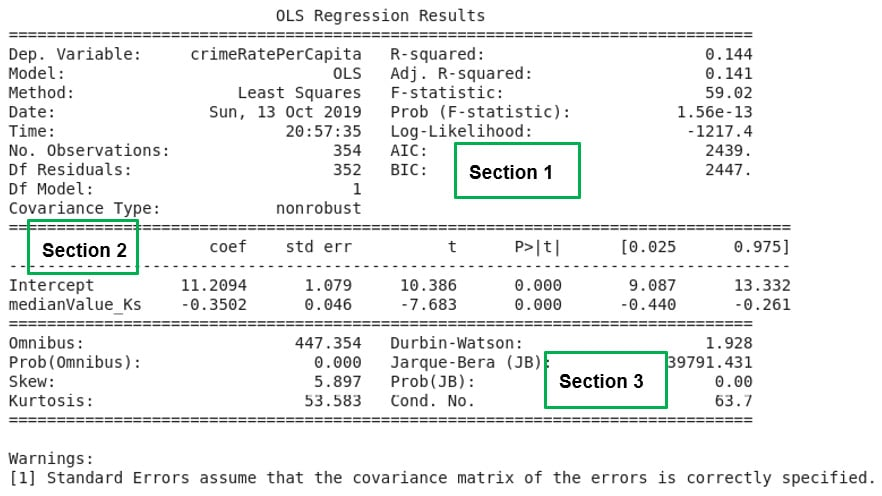Figure 2.15: A summary of the simple linear regression analysis results

If the exercise was correctly followed, then a model has been created with the `statsmodels` formula API. The `fit` method (`.fit()`) of the model object was called to fit the linear regression model to the data. What fitting here means is to estimate the regression coefficients (parameters) using the ordinary least squares method.

Note

To access the source code for this specific section, please refer to https://packt.live/3iJXXge.

You can also run this example online at https://packt.live/2YbZ54v.

## Analyzing the Model Summary

The `.fit` method provides many functions to explore its output. These include the `conf_int()`, `pvalues`, `tvalues`, and `summary()` parameters. With these functions, the parameters of the model, the confidence intervals, and the p-values and t-values for the analysis can be retrieved from the results. (The concept of p-values and t-values will be explained later in the chapter.)

The syntax simply involves following the dot notation, after the variable name containing the results, with the relevant function name – for example, `linearModelResult.conf_int()` will output the confidence interval values. The handiest of them all is the `summary()` function, which presents a table of all relevant results from the analysis.

In Figure 2.15, the output of the summary function used in Exercise 2.04, Fitting a Simple Linear Regression Model Using the Statsmodels formula API, is presented. The output of the summary function is divided, using double dashed lines, into three main sections.

In Chapter 9, Interpreting a Machine Learning Model, the results of the three sections will be treated in detail. However, it is important to comment on a few points here.

In the top-left corner of Section 1 in Figure 2.15, we find the dependent variable in the model (`Dep. Variable`) printed and `crimeRatePerCapita` is the value for Exercise 2.04, Fitting a Simple Linear Regression Model Using the Statsmodels formula API. A statistic named R-squared with a value of `0.144` for our model is also provided in Section 1. The R-squared value is calculated by Python as a fraction (`0.144`) but it is to be reported in percentages so the value for our model is `14.4%` The R-squared statistic provides a measure of how much of the variability in the dependent variable (`crimeRatePerCapita`), our model is able to explain. It can be interpreted as a measure of how well our model fits the dataset. In Section 2 of Figure 2.15, the intercept and the independent variable in our model is reported. The independent variable in our model is the median value of owner-occupied homes (`medianValue_Ks`).

In this same Section 2, just next to the intercept and the independent variable, is a column that reports the model coefficients (`coef`). The intercept and the coefficient of the independent variable are printed under the column labeled `coef` in the summary report that Python prints out. The intercept has a value of `11.2094` with the coefficient of the independent variable having a value of negative `0.3502` (`-0.3502`). If we choose to denote the dependent variable in our model (`crimeRatePerCapita`) as y and the independent variable (the median value of owner-occupied homes) as x, we have all the ingredients to write out the equation that defines our model.

Thus, y ≈ 11.2094 - 0.3502 x, is the equation for our model. In Chapter 9, Interpreting a Machine Learning Model, what this model means and how it can be used will be discussed in full.

## The Model Formula Language

Python is a very powerful language liked by many developers. Since the release of version 0.5.0 of statsmodels, Python now provides a very competitive option for statistical analysis and modeling rivaling R and SAS.

This includes what is commonly referred to as the R-style formula language, by which statistical models can be easily defined. Statsmodels implements the R-style formula language by using the `Patsy` Python library internally to convert formulas and data to the matrices that are used in model fitting.

Figure 2.16 summarizes the operators used to construct the `Patsy` formula strings and what they mean: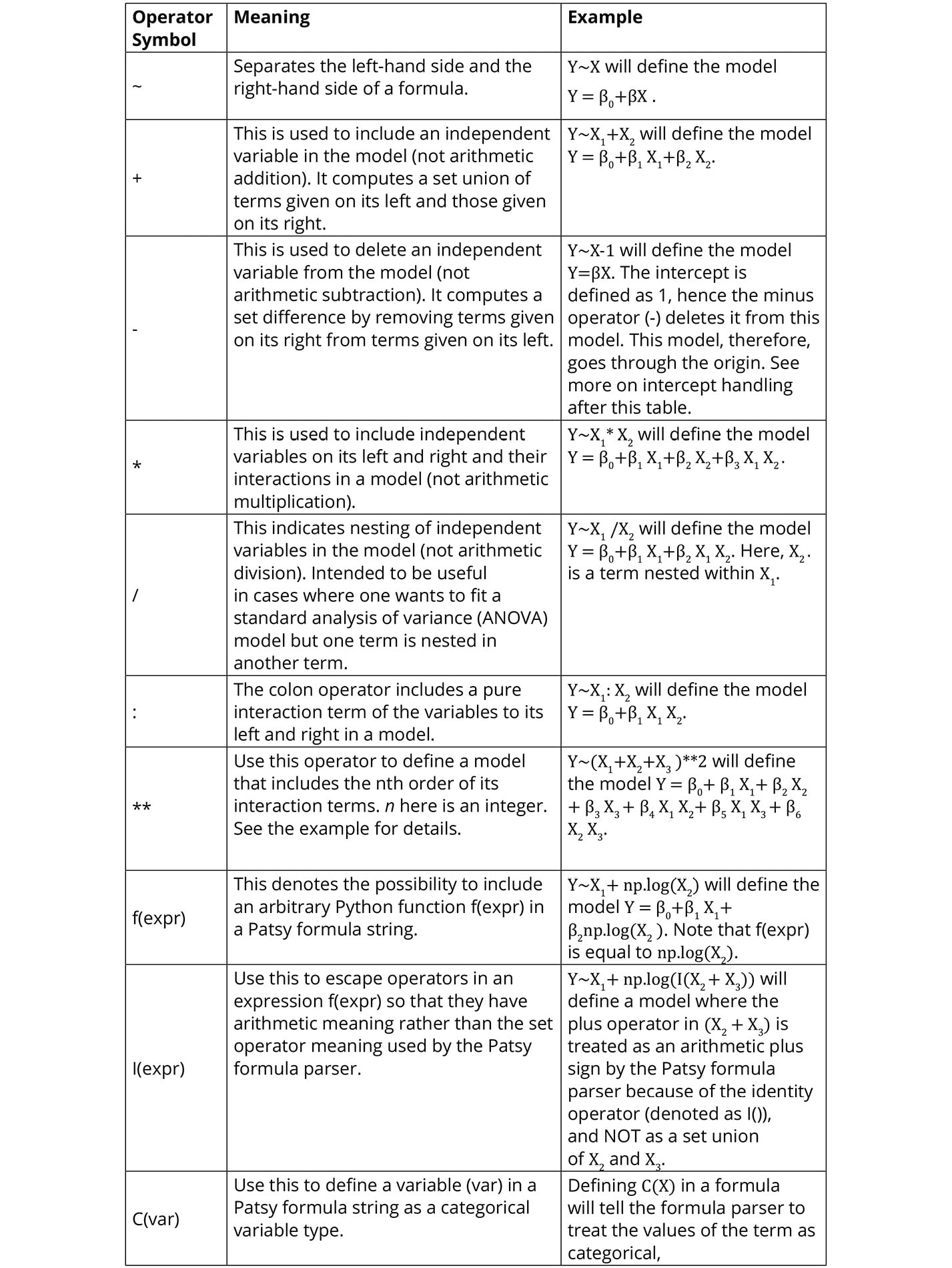Figure 2.16: A summary of the Patsy formula syntax and examples

## Intercept Handling

In patsy formula strings, `string 1` is used to define the intercept of a model. Because the intercept is needed most of the time, `string 1` is automatically included in every formula string definition. You don't have to include it in your string to specify the intercept. It is there invisibly. If you want to delete the intercept from your model, however, then you can subtract one (-1) from the formula string and that will define a model that passes through the origin. For compatibility with other statistical software, `Patsy` also allows the use of the string zero (0) and negative one (-1) to be used to exclude the intercept from a model. What this means is that, if you include 0 or -1 on the right-hand side of your formula string, your model will have no intercept.

## Activity 2.01: Fitting a Log-Linear Model Using the Statsmodels Formula API

You have seen how to use the statsmodels API to fit a linear regression model. In this activity, you are asked to fit a log-linear model. Your model should represent the relationship between the log-transformed dependent variable (log of crime rate per capita) and the median value of owner-occupied homes.

The steps to complete this activity are as follows:

1. Define a linear regression model and assign it to a variable. Remember to use the `log` function to transform the dependent variable in the formula string.
2. Call the `fit` method of the log-linear model instance and assign the results of the method to a variable.
3. Print a summary of the results and analyze the output.

Your output should look like the following figure: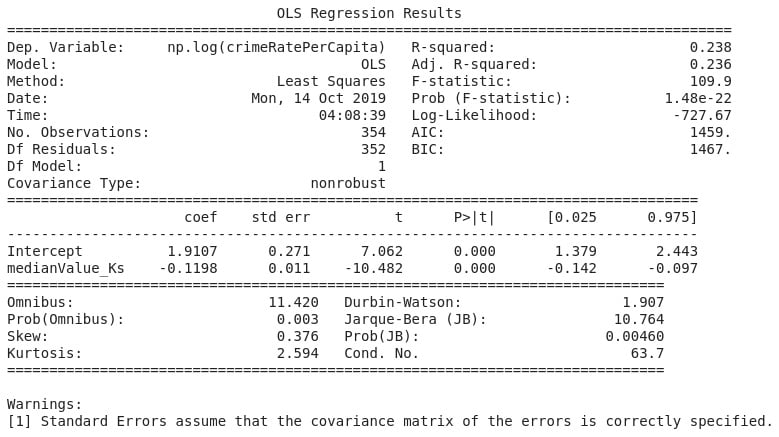Figure 2.17: A log-linear regression of crime rate per capita on the median value of owner-occupied homes

Note

The solution to this activity can be found here: https://packt.live/2GbJloz.

# Multiple Regression Analysis

In the exercises and activity so far, we have used only one independent variable in our regression analysis. In practice, as we have seen with the Boston Housing dataset, processes and phenomena of analytic interest are rarely influenced by only one feature. To be able to model the variability to a higher level of accuracy, therefore, it is necessary to investigate all the independent variables that may contribute significantly toward explaining the variability in the dependent variable. Multiple regression analysis is the method that is used to achieve this.

## Exercise 2.05: Fitting a Multiple Linear Regression Model Using the Statsmodels Formula API

In this exercise, we will be using the plus operator (`+`) in the `patsy` formula string to define a linear regression model that includes more than one independent variable.

To complete this activity, run the code in the following steps in your Colab notebook:

1. Open a new Colab notebook file and import the required packages.
```import statsmodels.formula.api as smf
import pandas as pd
from sklearn.model_selection import train_test_split```
2. Execute Step 2 to 11 from Exercise 2.01, Loading and Preparing the Data for Analysis.
3. Use the plus operator (`+`) of the Patsy formula language to define a linear model that regresses `crimeRatePerCapita` on `pctLowerStatus`, `radialHighwaysAccess`, `medianValue_Ks`, and `nitrixOxide_pp10m` and assign it to a variable named `multiLinearModel`. Use the Python line continuation symbol (`\`) to continue your code on a new line should you run out of space:
```multiLinearModel = smf.ols\
(formula = 'crimeRatePerCapita \
~ pctLowerStatus \
+ medianValue_Ks \
+ nitrixOxide_pp10m', \
data=train_data)```
4. Call the `fit` method of the model instance and assign the results of the method to a variable:
`multiLinearModResult = multiLinearModel.fit()`
5. Print a summary of the results stored the variable created in Step 3:
`print(multiLinearModResult.summary())`

The output is as follows: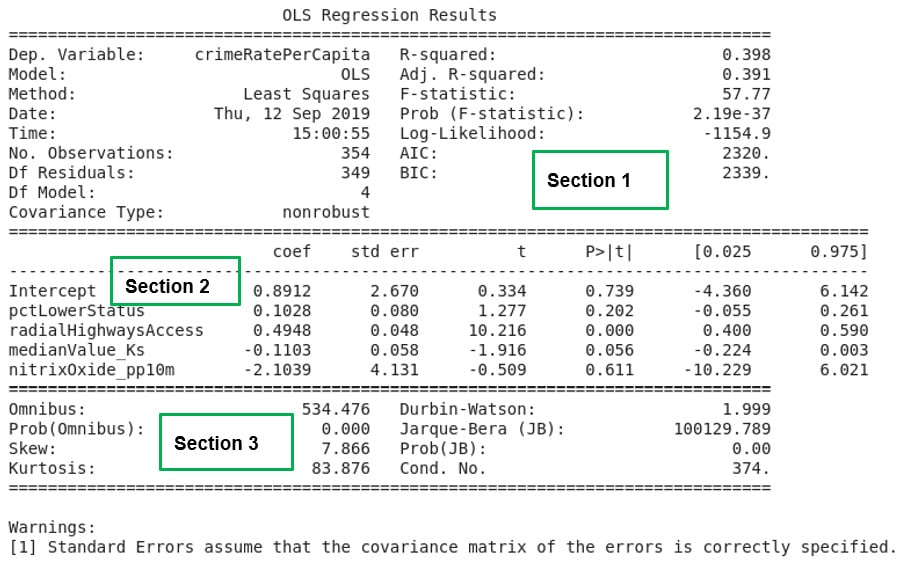Figure 2.18: A summary of multiple linear regression results

Note

To access the source code for this specific section, please refer to https://packt.live/34cJgOK.

You can also run this example online at https://packt.live/3h1CKOt.

If the exercise was correctly followed, Figure 2.18 will be the result of the analysis. In Activity 2.01, the R-squared statistic was used to assess the model for goodness of fit. When multiple independent variables are involved, the goodness of fit of the model created is assessed using the adjusted R-squared statistic.

The adjusted R-squared statistic considers the presence of the extra independent variables in the model and corrects for inflation of the goodness of fit measure of the model, which is just caused by the fact that more independent variables are being used to create the model.

The lesson we learn from this exercise is the improvement in the adjusted R-squared value in Section 1 of Figure 2.18. When only one independent variable was used to create a model that seeks to explain the variability in `crimeRatePerCapita` in Exercise 2.04, Fitting a Simple Linear Regression Model Using the Statsmodels formula API, the R-squared value calculated was only `14.4` percent. In this exercise, we used four independent variables. The model that was created improved the adjusted R-squared statistic to `39.1` percent, an increase of `24.7` percent.

We learn that the presence of independent variables that are correlated to a dependent variable can help explain the variability in the independent variable in a model. But it is clear that a considerable amount of variability, about `60.9` percent, in the dependent variable is still not explained by our model.

There is still room for improvement if we want a model that does a good job of explaining the variability we see in `crimeRatePerCapita`. In Section 2 of Figure 2.18, the intercept and all the independent variables in our model are listed together with their coefficients. If we denote `pctLowerStatus` by x1, `radialHighwaysAccess` by x2, `medianValue_Ks` by x3 , and `nitrixOxide_pp10m` by x4, a mathematical expression for the model created can be written as y ≈ 0.8912+0.1028x1+0.4948x2-0.1103x3-2.1039x4.

The expression just stated defines the model created in this exercise, and it is comparable to the expression for multiple linear regression provided in Figure 2.5 earlier.

# Assumptions of Regression Analysis

Due to the parametric nature of linear regression analysis, the method makes certain assumptions about the data it analyzes. When these assumptions are not met, the results of the regression analysis may be misleading to say the least. It is, therefore, necessary to check any analysis work to ensure the regression assumptions are not violated.

Let's review the main assumptions of linear regression analysis that we must ensure are met in order to develop a good model:

1. The relationship between the dependent and independent variables must be linear and additive.

This means that the relationship must be of the straight-line type, and if there are many independent variables involved, thus multiple linear regression, the weighted sum of these independent variables must be able to explain the variability in the dependent variable.

2. The residual terms (ϵi) must be normally distributed. This is so that the standard error of estimate is calculated correctly. This standard error of estimate statistic is used to calculate t-values, which, in turn, are used to make statistical significance decisions. So, if the standard error of estimate is wrong, the t-values will be wrong and so are the statistical significance decisions that follow on from the p-values. The t-values that are calculated using the standard error of estimate are also used to construct confidence intervals for the population regression parameters. If the standard error is wrong, then the confidence intervals will be wrong as well.
3. The residual terms (ϵi) must have constant variance (homoskedasticity). When this is not the case, we have the heteroskedasticity problem. This point refers to the variance of the residual terms. It is assumed to be constant. We assume that each data point in our regression analysis contributes equal explanation to the variability we are seeking to model. If some data points contribute more explanation than others, our regression line will be pulled toward the points with more information. The data points will not be equally scattered around our regression line. The error (variance) about the regression line, in that case, will not be constant.
4. The residual terms (ϵi) must not be correlated. When there is correlation in the residual terms, we have the problem known as autocorrelation. Knowing one residual term, must not give us any information about what the next residual term will be. Residual terms that are autocorrelated are unlikely to have a normal distribution.
5. There must not be correlation among the independent variables. When the independent variables are correlated among themselves, we have a problem called multicollinearity. This would lead to developing a model with coefficients that have values that depend on the presence of other independent variables. In other words, we will have a model that will change drastically should a particular independent variable be dropped from the model for example. A model like that will be inaccurate.

## Activity 2.02: Fitting a Multiple Log-Linear Regression Model

A log-linear regression model you developed earlier was able to explain about 24% of the variability in the transformed crime rate per capita variable (see the values in Figure 2.17). You are now asked to develop a log-linear multiple regression model that will likely explain 80% or more of the variability in the transformed dependent variable. You should use independent variables from the Boston Housing dataset that have a correlation coefficient of 0.4 or more.

You are also encouraged to include the interaction of these variables to order two in your model. You should produce graphs and data that show that your model satisfies the assumptions of linear regression.

The steps are as follows:

1. Define a linear regression model and assign it to a variable. Remember to use the `log` function to transform the dependent variable in the formula string, and also include more than one independent variable in your analysis.
2. Call the `fit` method of the model instance and assign the results of the method to a new variable.
3. Print a summary of the results and analyze your model.

Your output should appear as shown: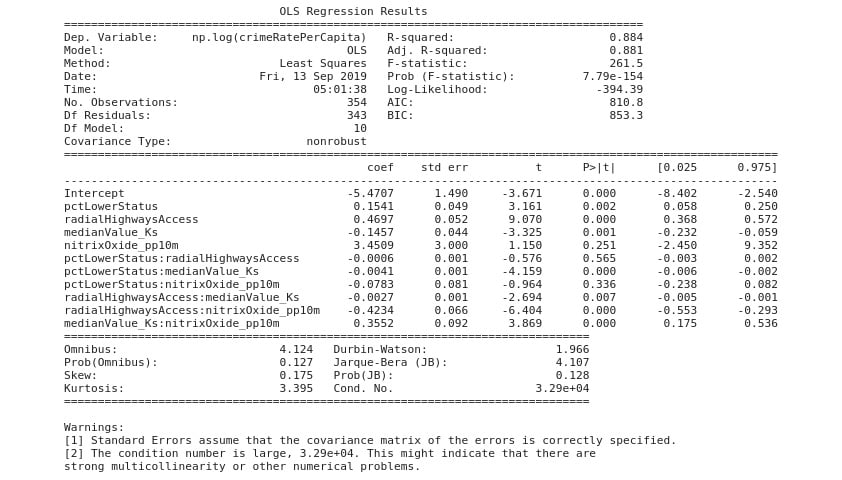Figure 2.19: Expected OLS results

Note

The solution to this activity can be found here: https://packt.live/2GbJloz.

# Explaining the Results of Regression Analysis

A primary objective of regression analysis is to find a model that explains the variability observed in a dependent variable of interest. It is, therefore, very important to have a quantity that measures how well a regression model explains this variability. The statistic that does this is called R-squared (R2). Sometimes, it is also called the coefficient of determination. To understand what it actually measures, we need to take a look at some other definitions.

The first of these is called the Total Sum of Squares (TSS). TSS gives us a measure of the total variance found in the dependent variable from its mean value.

The next quantity is called the Regression sum of squares (RSS). This gives us a measure of the amount of variability in the dependent variable that our model explains. If you imagine us creating a perfect model with no errors in prediction, then TSS will be equal to RSS. Our hypothetically perfect model will provide an explanation for all the variability we see in the dependent variable with respect to the mean value. In practice, this rarely happens. Instead, we create models that are not perfect, so RSS is less than TSS. The missing amount by which RSS falls short of TSS is the amount of variability in the dependent variable that our regression model is not able to explain. That quantity is the Error Sum of Squares (ESS), which is essentially the sum of the residual terms of our model.

R-squared is the ratio of RSS to TSS. This, therefore, gives us a percentage measure of how much variability our regression model is able to explain compared to the total variability in the dependent variable with respect to the mean. R2 will become smaller when RSS grows smaller and vice versa. In the case of simple linear regression where the independent variable is one, R2 is enough to decide the overall fit of the model to the data.

There is a problem, however, when it comes to multiple linear regression. The R2 is known to be sensitive to the addition of extra independent variables to the model, even if the independent variable is only slightly correlated to the dependent variable. Its addition will increase R2. Depending on R2 alone to make a decision between models defined for the same dependent variable will lead to chasing a complex model that has many independent variables in it. This complexity is not helpful practically. In fact, it may lead to a problem in modeling called overfitting.

To overcome this problem, the Adjusted R2 (denoted Adj. R-Squared on the output of statsmodels) is used to select between models defined for the same dependent variable. Adjusted R2 will increase only when the addition of an independent variable to the model contributes to explaining the variability in the dependent variable in a meaningful way.

In Activity 2.02, Fitting a Multiple Log-Linear Regression Model, our model explained 88 percent of the variability in the transformed dependent variable, which is really good. We started with simple models and worked to improve the fit of the models using different techniques. All the exercises and activities done in this chapter have pointed out that the regression analysis workflow is iterative. You start by plotting to get a visual picture and follow from there to improve upon the model you finally develop by using different techniques. Once a good model has been developed, the next step is to validate the model statistically before it can be used for making a prediction or acquiring insight for decision making. Next, let's discuss what validating the model statistically means.

## Regression Analysis Checks and Balances

In the preceding discussions, we used the R-squared and the Adjusted R-squared statistics to assess the goodness of fit of our models. While the R-squared statistic provides an estimate of the strength of the relationship between a model and the dependent variable(s), it does not provide a formal statistical hypothesis test for this relationship.

What do we mean by a formal statistical hypothesis test for a relationship between a dependent variable and some independent variable(s) in a model?

We must recall that, to say an independent variable has a relationship with a dependent variable in a model, the coefficient (β) of that independent variable in the regression model must not be zero (0). It is well and good to conduct a regression analysis with our Boston Housing dataset and find an independent variable (say the median value of owner-occupied homes) in our model to have a nonzero coefficient (β).

The question is will we (or someone else) find the median value of owner-occupied homes as having a nonzero coefficient (β), if we repeat this analysis using a different sample of Boston Housing dataset taken at different locations or times? Is the nonzero coefficient for the median value of owner-occupied homes, found in our analysis, specific to our sample dataset and zero for any other Boston Housing data sample that may be collected? Did we find the nonzero coefficient for the median value of owner-occupied homes by chance? These questions are what hypothesis tests seek to clarify. We cannot be a hundred percent sure that the nonzero coefficient (β) of an independent variable is by chance or not. But hypothesis testing gives a framework by which we can calculate the level of confidence where we can say that the nonzero coefficient (β) found in our analysis is not by chance. This is how it works.

We first agree a level of risk (α-value or α-risk or Type I error) that may exist that the nonzero coefficient (β) may have been found by chance. The idea is that we are happy to live with this level of risk of making the error or mistake of claiming that the coefficient (β) is nonzero when in fact it is zero.

In most practical analyses, the α-value is set at 0.05, which is 5 in percentage terms. When we subtract the α-risk from one (1-α) we have a measure of the level of confidence that we have that the nonzero coefficient (β) found in our analysis did not come about by chance. So, our confidence level is 95% at 5% α-value.

We then go ahead to calculate a probability value (usually called the p-value), which gives us a measure of the α-risk related to the coefficient (β) of interest in our model. We compare the p-value to our chosen α-risk, and if the p-value is less than the agreed α-risk, we reject the idea that the nonzero coefficient (β) was found by chance. This is because the risk of making a mistake of claiming the coefficient (β) is nonzero is within the acceptable limit we set for ourselves earlier.

Another way of stating that the nonzero coefficient (β) was NOT found by chance is to say that the coefficient (β) is statistically significant or that we reject the null hypothesis (the null hypothesis being that there is no relationship between the variables being studied). We apply these ideas of statistical significance to our models in two stages:

1. In stage one, we validate the model as a whole statistically.
2. In stage two, we validate the independent variables in our model individually for statistical significance.

## The F-test

The F-test is what validates the overall statistical significance of the strength of the relationship between a model and its dependent variables. If the p-value for the F-test is less than the chosen α-level (0.05, in our case), we reject the null hypothesis and conclude that the model is statistically significant overall.

When we fit a regression model, we generate an F-value. This value can be used to determine whether the test is statistically significant. In general, an increase in R2 increases the F-value. This means that the larger the F-value, the better the chances of the overall statistical significance of a model.

A good F-value is expected to be larger than one. The model in Figure 2.19 has an F-statistic value of 261.5, which is larger than one, and a p-value (Prob (F-statistic)) of approximately zero. The risk of making a mistake and rejecting the null hypothesis when we should not (known as a Type I error in hypothesis testing), is less than the 5% limit we chose to live with at the beginning of the hypothesis test. Because the p-value is less than 0.05, we reject the null hypothesis about our model in Figure 2.19. Therefore, we state that the model is statistically significant at the chosen 95% confidence level.

## The t-test

Once a model has been determined to be statistically significant globally, we can proceed to examine the significance of individual independent variables in the model. In Figure 2.19, the p-values (denoted `p>|t|` in Section 2) for the independent variables are provided. The p-values were calculated using the t-values also given on the summary results. The process is not different from what was just discussed for the global case. We compare the p-values to the 0.05 α-level. If an independent variable has a p-value of less than 0.05, the independent variable is statistically significant in our model in explaining the variability in the dependent variable. If the p-value is 0.05 or higher, the particular independent variable (or term) in our model is not statistically significant. What this means is that that term in our model does not contribute toward explaining the variability in our dependent variable statistically. A close inspection of Figure 2.19 shows that some of the terms have p-values larger than 0.05. These terms don't contribute in a statistically significant way of explaining the variability in our transformed dependent variable. To improve this model, those terms will have to be dropped and a new model tried. It is clear by this point that the process of building a regression model is truly iterative.

# Summary

This chapter introduced the topic of linear regression analysis using Python. We learned that regression analysis, in general, is a supervised machine learning or data science problem. We learned about the fundamentals of linear regression analysis, including the ideas behind the method of least squares. We also learned about how to use the pandas Python module to load and prepare data for exploration and analysis.

We explored how to create scatter graphs of bivariate data and how to fit a line of best fit through them. Along the way, we discovered the power of the statsmodels module in Python. We explored how to use it to define simple linear regression models and to solve the model for the relevant parameters. We also learned how to extend that to situations where the number of independent variables is more than one – multiple linear regressions. We investigated approaches by which we can transform a non-linear relation between a dependent and independent variable so that a non-linear problem can be handled using linear regression, introduced because of the transformation. We took a closer look at the statsmodels formula language. We learned how to use it to define a variety of linear models and to solve for their respective model parameters.

We continued to learn about the ideas underpinning model goodness of fit. We discussed the R-squared statistic as a measure of the goodness of fit for regression models. We followed our discussions with the basic concepts of statistical significance. We learned about how to validate a regression model globally using the F-statistic, which Python calculates for us. We also examined how to check for the statistical significance of individual model coefficients using t-tests and their associated p-values. We reviewed the assumptions of linear regression analysis and how they impact on the validity of any regression analysis work.

We will now move on from regression analysis, and Chapter 3, Binary Classification, and Chapter 4, Multiclass Classification with RandomForest, will discuss binary and multi-label classification, respectively. These chapters will introduce the techniques needed to handle supervised data science problems where the dependent variable is of the categorical data type.

Regression analysis will be revisited when the important topics of model performance improvement and interpretation are given a closer look later in the book. In Chapter 8, Hyperparameter Tuning, we will see how to use k-nearest neighbors and as another method for carrying out regression analysis. We will also be introduced to ridge regression, a linear regression method that is useful for situations where there are a large number of parameters.

• Anthony So

Anthony So is a renowned leader in data science. He has extensive experience in solving complex business problems using advanced analytics and AI in different industries including financial services, media, and telecommunications. He is currently the chief data officer of one of the most innovative fintech start-ups. He is also the author of several best-selling books on data science, machine learning, and deep learning. He has won multiple prizes at several hackathon competitions, such as Unearthed, GovHack, and Pepper Money. Anthony holds two master's degrees, one in computer science and the other in data science and innovation.

Browse publications by this author
• Thomas V. Joseph

Thomas V. Joseph is a data science practitioner, researcher, trainer, mentor, and writer with more than 19 years of experience. He has extensive experience in solving business problems using machine learning toolsets across multiple industry segments.

Browse publications by this author
• Robert Thas John

Robert Thas John is a Google developer expert in machine learning. His day job involves working as a data engineer on the Google Cloud Platform by building, training, and deploying large-scale machine learning models. He also makes decisions about how to store and process large amounts of data. He has more than 10 years of experience in building enterprise-grade solutions and working with data. He spends his free time learning or contributing to the developer community. He frequently travels to speak at technology events or to mentor developers. He also writes a blog on data science.

Browse publications by this author
• Andrew Worsley

Andrew David Worsley is an independent consultant and educator with expertise in the areas of machine learning, statistics, cloud computing, and artificial intelligence. He has practiced data science in several countries across a multitude of industries including retail, financial services, marketing, resources, and healthcare.

Browse publications by this author
• Dr. Samuel Asare

Dr. Samuel Asare is a professional engineer with enthusiasm for Python programming, research, and writing. He is highly skilled in applying data science methods to the extraction of useful insights from large data sets. He possesses solid skills in project management processes. Samuel has previously held positions, in industry and academia, as a process engineer and a lecturer of materials science and engineering respectively. Presently, he is pursuing his passion for solving industry problems, using data science methods, and writing.

Browse publications by this author
Latest Reviews (1 reviews total)
El contenido del libro me gusta bastante, y voy en el primer capitulo y cumple con mis espectativas
Recommended For YouThe Data Analysis Workshop

Learn how to analyze data using Python models with the help of real-world use cases and guidance from industry experts

By Gururajan Govindan and 2 moreThe Data Wrangling Workshop - Second Edition

A beginner’s guide to simplifying Extract, Transform, Load (ETL) processes with the help of hands-on tips, tricks, and best practices, in a fun and interactive way

By Brian Lipp and 2 moreThe Data Visualization Workshop

Explore a modern approach to visualizing data with Python and transform large real-world datasets into expressive visual graphics using this beginner-friendly workshop

By Mario Döbler and 1 moreMachine Learning for Algorithmic Trading - Second Edition

Leverage machine learning to design and back-test automated trading strategies for real-world markets using pandas, TA-Lib, scikit-learn, LightGBM, SpaCy, Gensim, TensorFlow 2, Zipline, backtrader, Alphalens, and pyfolio.

By Stefan Jansen